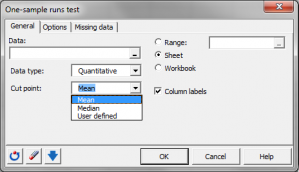# One sample runs test

The One sample runs test allows to check if a sequence of binary data is random or not. Run it in Excel using the XLSTAT add-on statistics software.## What is the one sample runs test

The one sample runs test is used to test whether a series of binary events can be considered as randomly distributed or not.

A run is a sequence of identical events, preceded and succeeded by different or no events. The runs test used here applies to binomial variables only. For example, in ABBABBB, we have 4 runs (A, BB, A, BBB).

The one sample runs test is used to test whether a series of binary events is randomly distributed or not.

## One sample runs test hypothesis

In the case of the two-tailed (or two-sided) test, the null (H0) and alternative (Ha) hypotheses are:

• H0: Data are randomly distributed.
• Ha: Data are not randomly distributed.

In the one-tailed case, you need to distinguish the left-tailed (or lower-tailed or lower one-sided) test and the right-tailed (or upper-tailed or upper one-sided) test. In the left-tailed test, the following hypotheses are used:

• H0: Data are randomly distributed.
• Ha: There is repulsion between the two types of events.

In the right-tailed test, the following hypotheses are used:

• H0: Data are randomly distributed.
• Ha: The two types of events are alternating.

### Expectation and variance of the one sample runs test

The expectation of the number of runs R is given by:

E(R) = 2mn/N

where m is the number of events of type 1, and n the number of events of type 2, and N is the total sample size.

The variance of the number of runs R is given by:

V(R) = 2mn(2mn – N)/[N²(N-1)]

The minimum value of R is always 2. The maximum value is given by 2Min(m, n) - t, where t is 1 if m=n, and 0 if not.

If r is the number of runs measured on the sample, it was shown by Wald and Wolfowitz that asymptotically, when m or n tend to infinity,

(r - E(R)) / √V(R) --> N(0,1)

where N(0,1) is the standard normal distribution.

### One sample runs test p-values

XLSTAT offers three ways to compute the p-values. You can compute the p-value based on:

• The exact distribution of R,
• The asymptotic distribution of R,
• An approximated distribution based on P Monte Carlo permutations. As the number of possible permutations is high (it is equal to N!), P must be set to a high value so that the approximation is fine.

## One sample runs test in XLSTAT

XLSTAT accepts as input, continuous data or binary categorical data. For continuous data, a cut-point must be chosen by the user so that the data are transformed into a binary sample.

A sample will be considered as randomly distributed if no particular structure can be identified. Extreme cases are repulsion, where you have all observations of one kind on the left, and all the remaining observations on the right, and alternation where the elements of the two kinds are alternating as much as possible. With the previous case, repulsion would give "AABBBBB" or "BBBBBAA", and alternation "BABABBB" or "BABBABB" or "BBABABB" or "BBABBAB" or "BBBABAB".### analice sus datos con xlstat

prueba gratuita de 14 días

Incluido en